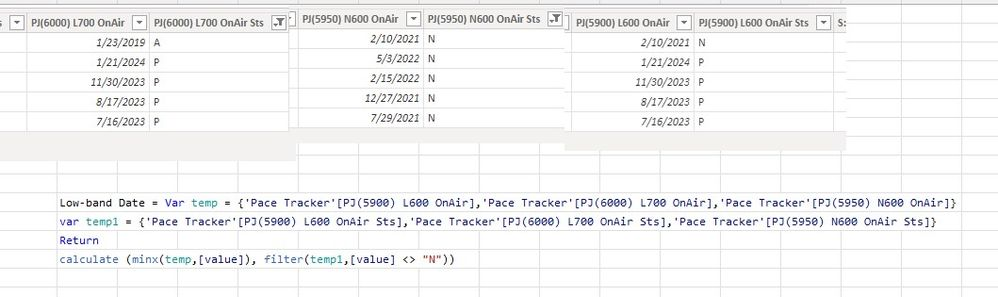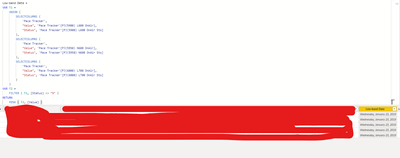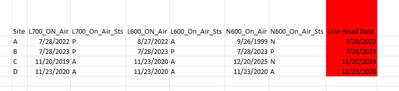cancel
Showing results for
Did you mean:Helper II

## var to calculate min with condition

I have few columns that have dates and with each date there are columns associated that has their status. I need to find the min of the dates where status is not equal to "N". I used the following formula but its giving me minimum of dates but not ignore the ones whos status is equal to N. Can someone help me to correct the formula, please?

Low-band Date = Var temp = {'Pace Tracker'[PJ(5900) L600 OnAir],'Pace Tracker'[PJ(6000) L700 OnAir],'Pace Tracker'[PJ(5950) N600 OnAir]}
var temp1 = {'Pace Tracker'[PJ(5900) L600 OnAir Sts],'Pace Tracker'[PJ(6000) L700 OnAir Sts],'Pace Tracker'[PJ(5950) N600 OnAir Sts]}
Return
calculate (minx(temp,[value]), filter(temp1,[value] <> "N"))1 ACCEPTED SOLUTIONSuper User

You are right. I misunderstood your requirement. You are trying to create a calculated column of the minimum date along the same row context given that the status of that date is not equal to "N".

``````Low-band Date =
VAR MinPJ5900 =
COALESCE (
IF (
'Pace Tracker'[PJ(5900) L600 OnAir Sts] <> "N",
'Pace Tracker'[PJ(5900) L600 OnAir]
),
DATE ( 3000, 1, 1 )
)
VAR MinPJ5950 =
COALESCE (
IF (
'Pace Tracker'[PJ(5950) N600 OnAir Sts] <> "N",
'Pace Tracker'[PJ(5950) N600 OnAir]
),
DATE ( 3000, 1, 1 )
)
VAR MinPJ6000 =
COALESCE (
IF (
'Pace Tracker'[PJ(6000) L700 OnAir Sts] <> "N",
'Pace Tracker'[PJ(6000) L700 OnAir]
),
DATE ( 3000, 1, 1 )
)
RETURN
MIN ( MIN ( MinPJ5900, MinPJ5950 ), MinPJ6000 )``````
16 REPLIES 16Super User

You may try

``````Low-band Date =
CALCULATE (
MIN (
MIN (
MIN ( 'Pace Tracker'[PJ(5900) L600 OnAir] ),
MIN ( 'Pace Tracker'[PJ(6000) L700 OnAir] )
),
MIN ( 'Pace Tracker'[PJ(5950) N600 OnAir] )
),
'Pace Tracker'[PJ(5900) L600 OnAir Sts] <> "N",
'Pace Tracker'[PJ(6000) L700 OnAir Sts] <> "N",
'Pace Tracker'[PJ(5950) N600 OnAir Sts] <> "N"
)``````Helper IIit says circular dependency. Also I may have more columns for few cases as well. the formula is working fine to calculate the min but when I am putting condition not to use status "N" for all then the dates doesn't work.Super User

Are you creating a mesure of a calculated column?Helper II

I have multiple columns of dates and their status  for each date column

I am trying to pull min for dates for each row where status for the dates is not equal to "N". and that is not a measure, its will be a new column.Super User

Yes but applying the filter in this way won't work. If you have too many columns then it would be wise to unpivot them using power query then the DAX would be much more simple. Otherwise please try the following nested MIN. YOu can add one more VAR and one more MIN in case you need to include one more column

``````Low-band Date =
VAR MinPJ5900 =
CALCULATE (
MIN ( 'Pace Tracker'[PJ(5900) L600 OnAir] ),
'Pace Tracker'[PJ(5900) L600 OnAir Sts] <> "N"
)
VAR MinPJ5950 =
CALCULATE (
MIN ( 'Pace Tracker'[PJ(5950) N600 OnAir] ),
'Pace Tracker'[PJ(5950) N600 OnAir Sts] <> "N"
)
VAR MinPJ6000 =
CALCULATE (
MIN ( 'Pace Tracker'[PJ(6000) L700 OnAir] ),
'Pace Tracker'[PJ(6000) L700 OnAir Sts] <> "N"
)
RETURN
MIN ( MIN ( MinPJ5900, MinPJ5950 ), MinPJ6000 )``````Helper IIits giving me same error again and for min I am trying to find that for the row not the min of the column, I think the min that you are calculating in this is for the column. I am trying to find the minimum on the dates for that row where that row date status is <> N for that dateSuper User

This is what the code is trying to calculate but seems this is creating a circular dependancy. Let's try another approach

``````Low-band Date =
VAR T1 =
UNION (
SELECTCOLUMNS (
'Pace Tracker',
"Value", 'Pace Tracker'[PJ(5900) L600 OnAir],
"Status", 'Pace Tracker'[PJ(5900) L600 OnAir Sts]
),
SELECTCOLUMNS (
'Pace Tracker',
"Value", 'Pace Tracker'[PJ(5950) N600 OnAir],
"Status", 'Pace Tracker'[PJ(5950) N600 OnAir Sts]
),
SELECTCOLUMNS (
'Pace Tracker',
"Value", 'Pace Tracker'[PJ(6000) L700 OnAir],
"Status", 'Pace Tracker'[PJ(6000) L700 OnAir Sts]
)
)
VAR T2 =
FILTER ( T1, [Status] <> "N" )
RETURN
MINX ( T2, [Value] )``````Helper IIits giving me value for the min of all rows, not per row levelSuper User

You are right. I misunderstood your requirement. You are trying to create a calculated column of the minimum date along the same row context given that the status of that date is not equal to "N".

``````Low-band Date =
VAR MinPJ5900 =
COALESCE (
IF (
'Pace Tracker'[PJ(5900) L600 OnAir Sts] <> "N",
'Pace Tracker'[PJ(5900) L600 OnAir]
),
DATE ( 3000, 1, 1 )
)
VAR MinPJ5950 =
COALESCE (
IF (
'Pace Tracker'[PJ(5950) N600 OnAir Sts] <> "N",
'Pace Tracker'[PJ(5950) N600 OnAir]
),
DATE ( 3000, 1, 1 )
)
VAR MinPJ6000 =
COALESCE (
IF (
'Pace Tracker'[PJ(6000) L700 OnAir Sts] <> "N",
'Pace Tracker'[PJ(6000) L700 OnAir]
),
DATE ( 3000, 1, 1 )
)
RETURN
MIN ( MIN ( MinPJ5900, MinPJ5950 ), MinPJ6000 )``````Helper II

that did worked now. thanks a lot. Can you explain what does coalesce and date (3000,1,1) will do? I just want to make sure when I am doing that to more columns it still work.

Regards,Super User

This is just to return a date in the future incase the if condition is not stisfied. As otherwise IF will return Blnak and then MIN will return Blank as minimum value.Helper II

Ok thanks a lot for all your helpSuper User

Please consider marking the correct solution as acceptable. Thank you.Helper II

here is some sample data and in red this is what I wan to calculate. Ignore the date where there is "N' for its stauts and calculate the minimum for the remaining on the specific rowHelper II
its giving me error that can't find table temp, the one i created to get onair dates from different columns

Low-band Date = Var temp = {'Pace Tracker'[PJ(5900) L600 OnAir],'Pace Tracker'[PJ(6000) L700 OnAir],'Pace Tracker'[PJ(5950) N600 OnAir]}
var temp1 = {'Pace Tracker'[PJ(5900) L600 OnAir Sts],'Pace Tracker'[PJ(6000) L700 OnAir Sts],'Pace Tracker'[PJ(5950) N600 OnAir Sts]}
Return
minx(FILTER(temp1, [Value] <> "N"),temp[value])Super User

@aahmad28 , if they are multiple tables append then and then try to have measure like

minx(filter(Table, [value] <> "N"), Table[Date])

!! Microsoft Fabric !!
Microsoft Power BI Learning Resources, 2023 !!
Learn Power BI - Full Course with Dec-2022, with Window, Index, Offset, 100+ Topics !!
Did I answer your question? Mark my post as a solution! Appreciate your Kudos !! Proud to be a Super User! !!